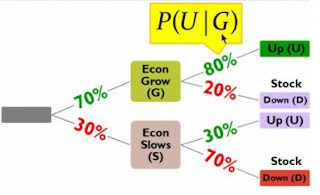## Wednesday, April 29, 2009

### Bayes' Formula

Author's Note: This is a great video for teaching Bayes' Theorem in its simplest form.

Summary
In order to illustrate the utility of Bayes’ Theorem, the author draws upon two simple scenarios. First, suppose someone faces the decision of needing to choose between three doors. If the person making the decision does not have any prior knowledge about the situation, the scenario creates an unconditional probability. But, once the person receives new information about the scenario, the rational person should reconsider his/her decision and subsequent probabilities.

Bayes’ Theorem is about the introduction of new information used to adjust probabilities and create conditional probabilities. In the formula, P(G/U), P is the probability that G will occur, if U happens.

To illustrate the application of Bayes’ Theorem and conditional probabilities, the author illustrates a second scenario. Pretend that there is a 70% probability that the economy will grow and a 30% probability that the economy will slow (an unconditional probability). The author owns a stock that has an 80% chance of increasing if the economy grows. That same stock, however, only has a 30% chance of increasing if the economy slows. The 80% and the second 30% are conditional probabilities; they are based on the condition that the economy will grow or slow.The author can then determine the scenario’s four conditional probabilities:
1) What is the probability that the economy will grow and the stock will increase?
2) What is the probability that the economy will grow and the stock will decrease?
3) What is the probability that the economy will slow and the stock will increase?
4) What is the probability that the economy will slow and the stock will decrease?

To answer these questions and determine their probabilities, the author uses the equation: P(UG) = P(U/G)P(G). Notice that this equation is longer than the first because this one incorporates two conditions: the economy will grow/slow and the stock will increase/decrease.

#### 1 comment:

1.That was helpful. Especially your use of example helped in understanding it.